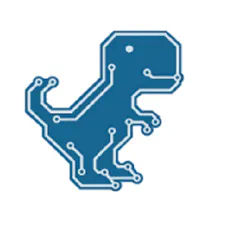# Ultrasonic Radar Can Detect Multiple Objects at Each Ping

With a small modification of the ultrasonic sensor module, this radar can detect multiple objects at each ping.

BeginnerFull instructions provided2,242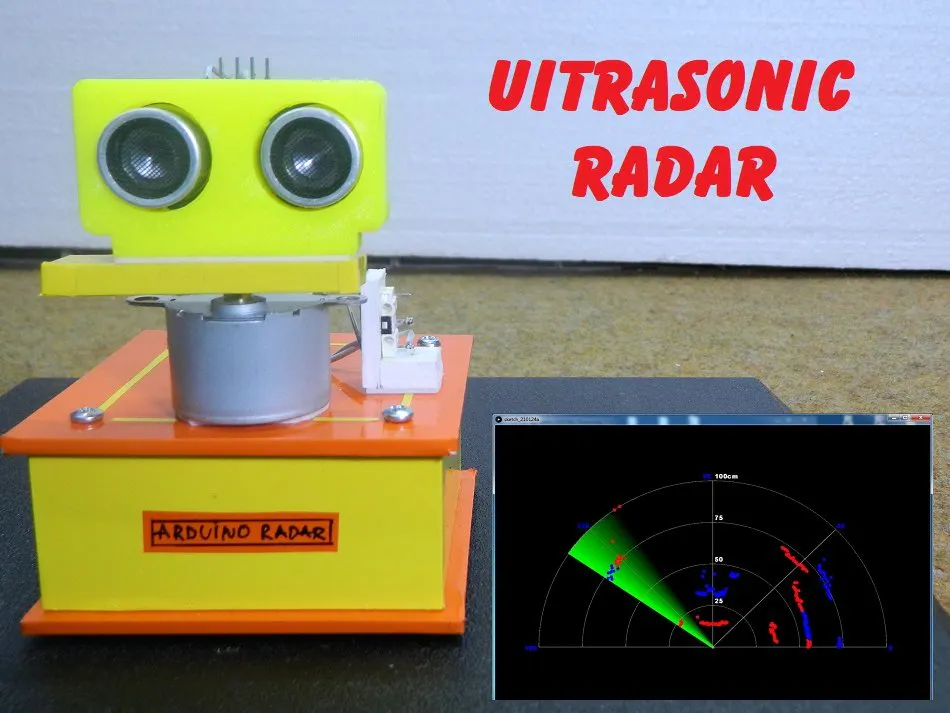## Things used in this project

### Hardware componentsArduino Nano R3
×1
 28byj-48 Stepper motor
×1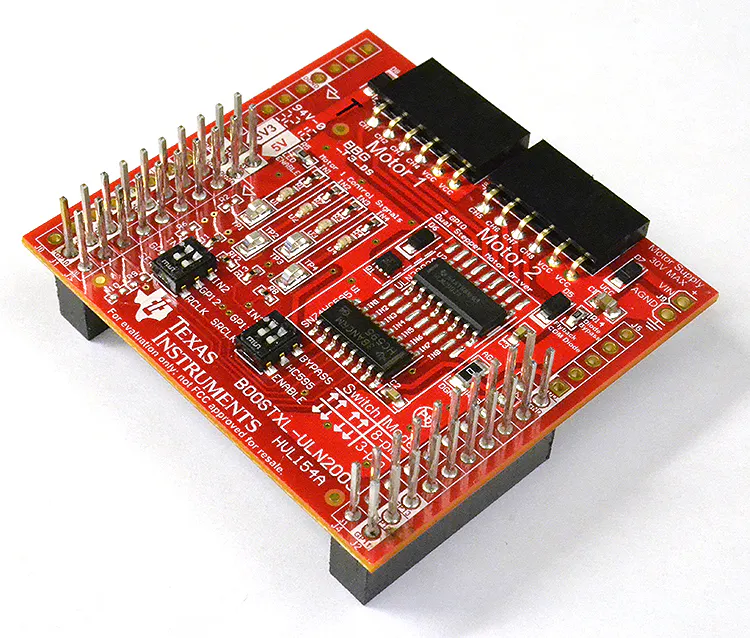Texas Instruments BOOSTXL-ULN2003 ULN2003A Dual Stepper Motor BoosterPack
×1Ultrasonic Sensor - HC-SR04 (Generic)
×1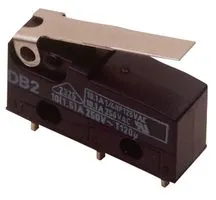Microswitch, IP67
×1

### Software apps and online servicesArduino IDE

### Hand tools and fabrication machinesSoldering iron (generic)

## Schematics

### Schematic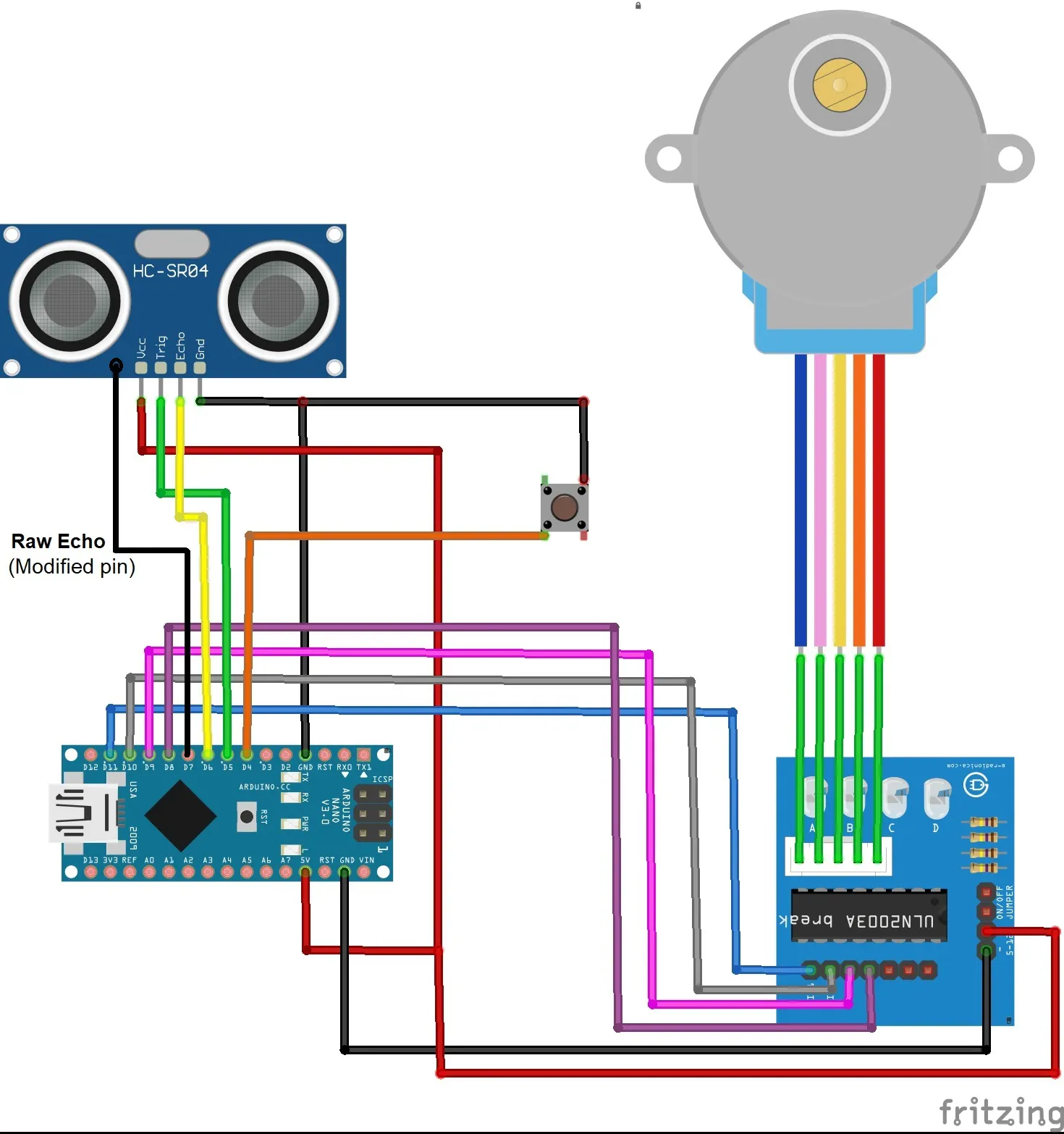## Code

### Arduino Code

C/C++
```// ======================
// globals
// ======================

// ----- serial port
#define Baud_rate 9600          //communication speed

// ----- serial input
char Char;

// ----- micro-switch
#define Micro_switch 4
bool Switch_present = true;       //no micro-switch = false

// ----- HC-SR04 / HY-SRF05 ultrasonic transducer
#define Trig 5
#define Echo 6
#define RawEcho 7                 //extra wire connected to pin 10 (see text)

// ----- motor controller definitions
/*
Connect your motor controller pins IN1..IN4 to the following Arduino pins.
The Arduino "talks" directly to controller pins IN1..IN4 via PORTB.
*/
#define IN4  8
#define IN3  9
#define IN2  10
#define IN1  11

// ----- motor definitions
/*
The 28BJY-48 5V DC motor has a "stride Angle" of 5.625/64 degrees per step.
One complete revolution therefore requires 360/5.625*64 equals 4096 steps.

This motor may be run using one of three possible modes:
1. Wave-stepping: lowest torque, max speed, coarse movement
2. Full-stepping: highest torque, max speed, coarse movement
3. Half-stepping: medium torque, half speed, smooth movement

From experiment "full-stepping" (which has the most torque) requires a minimum
delay of 2mS for reliable starting, whereas "half-stepping" only requires 1mS.
As a result the rotation speeds are the same. Since there is no speed advantage
"half-stepping" has been chosen for smoothness of rotation and reliable starting.

The required "half-stepping" motor pattern to achieve this is shown below.
*/
// ----- motor pattern
byte Motor =                  //half-stepping
{ B00001000,
B00001100,
B00000100,
B00000110,
B00000010,
B00000011,
B00000001,
B00001001
};

int Index = 0;                  //Motor[] array index
int Step_counter = 0;           //180 degrees requires 2048 steps
unsigned long Delay = 2;        //give motor shaft time to move
byte Pattern;                   //Motor[] pattern

// ----- acoustic "radar" display data
int Azimuth = 0;                //Azimuth (PI/128 radians) measured CCW from reference
int Distance1 = 0;
int Distance2 = 0;
int Direction = 0;              //counter-clockwise=0, clockwise=1

unsigned long
Speed_of_rotation = 30;         //controls beam rotation: 1 = fastest

// ======================
// setup
// ======================
void setup()
{
// ----- configure serial port
Serial.begin(Baud_rate);

// ----- configure micro-switch
pinMode(Micro_switch, INPUT_PULLUP);  //"wire-OR" normally HIGH

// ----- configure arduino pinouts
pinMode(Echo, INPUT);               //make Echo pin an input
pinMode(RawEcho, INPUT);            //make RawEcho pin an input
pinMode(Trig, OUTPUT);              //set Trig pin LOW
digitalWrite(Trig, LOW);

// ----- configure stepper motor
Pattern = DDRB;                       // get PORTB data directions
Pattern = Pattern | B00001111;        // preserve MSN data direction &
DDRB = Pattern;                       // make pins 8,9,10,11 outputs

// ----- rotate beam to start-up position
if (Switch_present)
{
home();
}

// ----- attach the graphics display
connect_to_display();                 //connect to the display
}

// ======================
// loop
// ======================
void loop()
{
// ----- has the display asked for data
if (Serial.available() > 0)
{

// ----- send data to display whenever a send character ('S') is received
if (Char == 'S')
{
// ----- measure distances
measure();

// ----- rotate beam to next ping position
rotate();

// ----- send the results to the display
Serial.print(Azimuth);
Serial.print(',');
Serial.print(Distance1);
Serial.print(',');
Serial.print(Distance2);
Serial.print(',');
Serial.println(Direction);

delay(Speed_of_rotation);         //slows rotational speed
}
}
}

// ===============================
// connect to graphics display
// ===============================
void connect_to_display()
{
while (Serial.available() <= 0)
{
// ----- keep sending synch ('S') until the display responds
Serial.println("S");
delay(250);
}
}

// ===============================
// measure distances
// ===============================
void measure()
{
// ----- locals
unsigned long start_time;           //microseconds
unsigned long finish_time;          //microseconds
unsigned long time_taken;           //microseconds
unsigned long timeout;              //microseconds
unsigned long pause;                //microseconds
boolean flag;

// ----- generate 10uS start pulse
digitalWrite(Trig, HIGH);
delayMicroseconds(10);
digitalWrite(Trig, LOW);

// ----- wait for pulse(s) to be sent
start_time = micros();

timeout = start_time + 12000;               //set timeout radius to 2 meters

// ----- measure first object distance
flag = false;
while (!flag)
{
if (!digitalRead(Echo)) flag = true;      //exit loop if object detected
if (timeout < micros()) flag = true;      //exit loop if timeout exceeded
}
finish_time = micros();

// ----- calculate first object distance(cm)
time_taken = finish_time - start_time;
Distance1 = ((float)time_taken) / 59;

// ----- wait for first object echo to finish
pause = finish_time + 1000;                 //1000uS means 17cm closest object spacing
while (pause > micros());                   //wait 1000uS

// ----- measure second object distance
flag = false;
while (!flag)                               //wait for high
{
if (digitalRead(RawEcho)) flag = true;    //exit loop if object dectected
if (timeout < micros()) flag = true;      //exit loop if timeout exceeded
}
finish_time = micros();

// ----- calculate second object distance (cm)
time_taken = finish_time - start_time;
Distance2 = ((float)time_taken) / 59;
}

// ===============================
// rotate motor to next ping position
// ===============================
void rotate()
{
// ----- counter-clockwise scan
if (Direction == 0)
{
for (int i = 0; i < 8; i++)
{
// ----- rotate motor to next ping position
Index = Step_counter % 8;                 //calculate array index
Pattern = PORTB;                          //get current motor pattern
Pattern = Pattern & B11110000;            //preserve MSN
Pattern = Pattern | Motor[Index];         //create new motor pattern
PORTB = Pattern;                          //send new pattern to motor
Step_counter++;
delay(Delay);                             //controls motor speed (fastest=1)
}

// ----- loop control
Azimuth++;
if (Azimuth > 256)
{
Azimuth = 256;
Direction = 1;
Step_counter = 2048;
}
}
else
{
// ----- clockwise scan
for (int i = 0; i < 8; i++)
{
// ----- rotate motor to next ping position
Index = Step_counter % 8;                 //calculate array index
Pattern = PORTB;                          //get current motor pattern
Pattern = Pattern & B11110000;            //preserve MSN
Pattern = Pattern | Motor[Index];         //create new motor pattern
PORTB = Pattern;                          //send new pattern to motor
Step_counter--;
delay(Delay);                             //controls motor speed (fastest=1)
}

// ----- loop control
Azimuth--;                                  //decrement Azimuth every 8 steps
if (Azimuth < 0)
{
Azimuth = 0;
Direction = 0;
Step_counter = 0;
}
}
}

// ===============================
// find zero position for beam
// ===============================
void home()
{
// ----- rotate clockwise until limit switch operates
Step_counter = 2048;
{
Index = Step_counter % 8;                 //calculate array index
Pattern = PORTB;                          //get current motor pattern
Pattern = Pattern & B11110000;            //preserve MSN
Pattern = Pattern | Motor[Index];         //create new motor pattern
PORTB = Pattern;                          //send new pattern to motor
Step_counter--;
delay(Delay);                             //controls motor speed (fastest=1)
}

// ----- back off slightly
/*
Keep clear of limit switch during normal scans
*/
Step_counter = 0;
for (int i = 0; i < 250; i++)
{
Index = Step_counter % 8;                 //calculate array index
Pattern = PORTB;                          //get current motor pattern
Pattern = Pattern & B11110000;            //preserve MSN
Pattern = Pattern | Motor[Index];         //create new motor pattern
PORTB = Pattern;                          //send new pattern to motor
Step_counter++;
delay(Delay);                             //controls motor speed (fastest=1)
}

// ----- reset the step counter
Step_counter = 0;
}
```

### Processing Code

C/C++
```// ======================
// globals
// ======================

// ----- serial port
import processing.serial.*;             //import the serial library
Serial myPort;                          //the Serial port object
final int Baud_rate = 9600;           //communication speed
String Input_string;                    //for incoming data
boolean Connected = false;              //flag

// ----- display graphics
PGraphics Canvas;                       //name of drawing area to be created
PFont myFont;                           //name of font to be created

// ----- ultrasonic beam
int [][] Ping = new int ;       //257 rows of 2 columns
int Direction = 0;                      //scan direction: true=CW, false=CCW

// ======================
// setup
// ======================
void setup()
{
// ----- image window
//size(900, 600, P3D);                                       //P3D parameter allows rotation around Z-axis
//size(1200, 800, P3D);                                       //P3D parameter allows rotation around Z-axis
size(1050, 700, P3D);
// ----- create a drawing area for fading the beam
Canvas = createGraphics(width, height);

// ------ create the screen font
myFont = createFont("Arial Black", 20);

// ----- initialize the serial port
printArray(Serial.list());                                //lists your COM ports on screen
myPort = new Serial(this, Serial.list(), Baud_rate);
myPort.bufferUntil('\n');
}

// ======================
// draw
// ======================
void draw()

{
// ----- define colors, scale, & text
background(0);                                    //black background
textFont(myFont, 20);                            //specify font to be used

// ----- draw beam on its own canvas
Canvas.beginDraw();
Canvas.translate(width/2, height*0.8);            //beam origin
Canvas.stroke(0, 255, 0);                         //green beam
Canvas.strokeWeight(7);                           //set beam-width
Canvas.scale(0.8);                                //think 100% but scale 80%
Canvas.rotate(-Azimuth*PI/256);                   //rotate "sheet of paper" but
Canvas.line(0, 0, width/2, 0);                    //think horizontal lines
Canvas.endDraw();

// ----- draw the graticule
draw_graticule();

// ----- plot CCW data
if (Direction == 0)
{
for (int i=0; i<Azimuth+1; i++)
{
plot_data(i);                                    //plot data points BELOW azimuth

}
}

// ----- plot CW data
if (Direction == 1)
{
for (int i=Azimuth; i<257; i++)
{
plot_data(i);                                   //plot data points ABOVE azimuth
}
}

// ----- superimpose beam over the display canvas
image(Canvas, 0, 0);

fadeGraphics(Canvas, 5);                           //the number controls the beam width
}

// =======================
// serial event  (called with each Arduino data string)
// =======================
void serialEvent(Serial myPort)
{
// ----- wait for a line-feed

// ----- validate
if (Input_string != null)
{
// ----- trim whitespace
Input_string = trim(Input_string);
println(Input_string);

// ----- make contact
if (Connected == false)
{
if (Input_string.equals("S"))
{
// ----- set flag

// ----- request data
myPort.write("S");         //request data
}
} else
// ----- send data
{
Input_string = trim(Input_string);              //remove leading/trailing whitespace
println(Input_string);

int[] values = int(split(Input_string, ','));
Azimuth = values;
Ping[Azimuth] = values;
Ping[Azimuth] = values;
Direction = values;

println(Azimuth);
println(Ping[Azimuth]);
println(Ping[Azimuth]);
println(Direction);

myPort.write("S");
}
}
}

// =======================
// draw graticule (horizontal text)
// =======================
void draw_graticule()
{
// ----- setup
pushMatrix();                                     //save screen parameters
translate(width/2, height*0.8);                   //move the origin
scale(0.8);                                       //scale everything 80%

// ----- draw the arcs
stroke(128);                                      //use gray lines
arc(0, 0, width, -width, 0, PI, CHORD);           //CHORD draws the baseline
arc(0, 0, width*0.75, -width*0.75, 0, PI, OPEN);
arc(0, 0, width*0.5, -width*0.5, 0, PI, OPEN);
arc(0, 0, width*0.25, -width*0.25, 0, PI, OPEN);

pushMatrix();                                     //save screen parameters
stroke(128);                                      //use gray lines
line(0, 0, width/2, 0);                           //draw line at 45
line(0, 0, width/2, 0);                           //draw line at 90
line(0, 0, width/2, 0);                           //draw line at 135
popMatrix();                                      //restore screen parameters

fill(0, 0, 255);                                  //blue text
textAlign(LEFT, CENTER);
text("0", width/2+5, 0);                          //"0" degrees

textAlign(LEFT, BOTTOM);
text("45", width*0.35+5, -width*0.35);             //"45" degrees

textAlign(RIGHT, BOTTOM);
text("90", -5, -width/2);                         //"90" degrees

textAlign(RIGHT, BOTTOM);
text("135", -width*0.35-5, -width*0.35);          //"135" degrees

textAlign(RIGHT, CENTER);
text("180", -width/2-5, 0);                       //"180" degrees

// ----- label the arcs
fill(255);                                        //light gray text
textAlign(LEFT, BOTTOM);
text("100cm", +5, -width/2);                      //"100cm"
text("75", +5, -width/2*0.75);                    //"75cm"
text("50", +5, -width/2*0.5);                     //"50cm"
text("25", +5, -width/2*0.25);                    //"25cm"

// ----- restore properties
strokeWeight(1);
fill(0);                                          //white
stroke(255);                                      //black
scale(1.0);
popMatrix();                                      //restore screen parameters
}

// =======================
// plot data
// =======================
void plot_data(int index)
{
// ----- setup
pushMatrix();                          //save screen parameters
translate(width/2, height*0.8);        //move the origin
scale(0.8);

// ----- plot array contents
rotateZ(-index*PI/256);                //rotate the display coordinates
strokeWeight(5);                       //set data size

stroke(255, 0, 0);                     //set data1 color to red
if (Ping[index]>100) Ping[index] = 1000;      //hide by printing off-screen
ellipse(width/2*Ping[index]/100, 0, 5, 5);      //plot data1

stroke(0, 0, 255);                     //set data2 color to blue
if (Ping[index]>100) Ping[index] = 1000;      //hide by printing off-screen
ellipse(width/2*Ping[index]/100, 0, 5, 5);      //plot data2

// ----- restore defaults
strokeWeight(1);
stroke(0);
popMatrix();                           //restore screen parameters
}

// =======================
// =======================
/*
This fadeGraphics() routine was found at
*/
{
c.beginDraw();

// ----- iterate over pixels
for (int i =0; i<c.pixels.length; i++)
{
// ----- get alpha value
int alpha = (c.pixels[i] >> 24) & 0xFF ;

// ----- reduce alpha value

// ----- assign color with new alpha-value
c.pixels[i] = alpha<<24 | (c.pixels[i]) & 0xFFFFFF ;
}

Canvas.updatePixels();
Canvas.endDraw();
}
```

## Credits

### Mirko Pavleski

20 projects • 524 followers